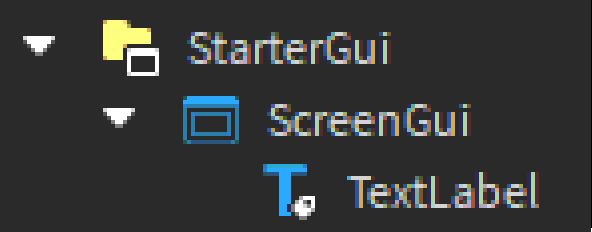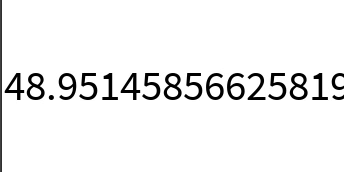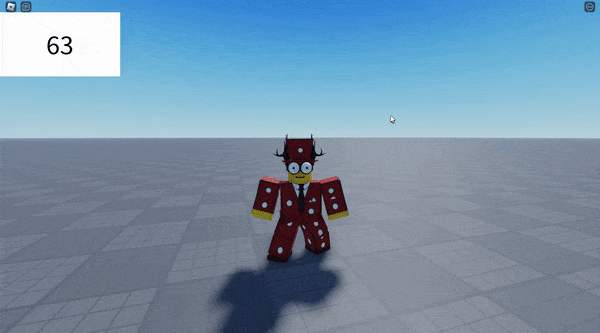# How to make a simple fps counter!

ello, this is my first community tutorial so please let me know if i should change anything!

here’s a step by step guide on how to code a simple fps counter!

firstly, you need to have a screengui with a textlabel inside to display the fps, like this:note: you don’t need to put any text inside the textlabel, but personally i like to add a placeholder text

now let’s get to the coding!

let’s add a localscript in the textlabel and write some code:

``````local runservice = game:GetService("RunService")

local textlabel = script.Parent

local fps
``````

we’re gonna use runservice for the `frametime`, and we’ll make an empty fps variable for later

now, let’s make a renderstepped function:

``````runservice.RenderStepped:Connect(function(frametime)
--we gon write some stuff here in a second
end)
``````

what the `frametime` variable means is the time passed in seconds since the last frame, we’ll use this to get the actual fps

now to change the text, we’ll have to first get the actual fps!
to do this, we gonna do some quick maths:

``````runservice.RenderStepped:Connect(function(frametime)
fps = 1/frametime
end)
``````

so lemme explain how this works:

let’s say you have 60 fps; that means a frame gets rendered every 0.0166… seconds (which is the frametime), and the way we calculate this is by doing 1/fps

now if we do it the other way around, and do 1/0.0166… then we’ll get 60 fps

so now the `fps` variable is equal to the frames per second, great!

now all we have to do is change the text to the fps:

``````runservice.RenderStepped:Connect(function(frametime)
fps = 1/frametime
textlabel.Text = tostring(fps)
end)
``````

now if we test it, it does work but you have likely noticed the huge amount of decimals in the counter:and of course, we don’t want this, so we’ll use math.floor!

``````textlabel.Text = tostring(math.floor(fps))
``````

math.floor basically takes out all of those ugly decimals and makes the number nice and clean!

now if we test, we’ll have a working fps counter!full code:
``````local runservice = game:GetService("RunService")

local textlabel = script.Parent

local fps

runservice.RenderStepped:Connect(function(frametime)

fps = 1/frametime

textlabel.Text = tostring(math.floor(fps))

end)
``````

hope this guide taught you something new! once again, let me know if i should change anything in this post, and have a great day!

9 Likes

thanks! i was finding on how to make one.

2 Likes

Simplified:

``````local runService = game:GetService("RunService")
runService.RenderStepped:Connect(function(deltaTime)
script.Parent.Text = tostring(math.floor(1 / deltaTime))
end)
``````
1 Like

I was really hoping it was going to be more accurate than this…
In the video, your fps shows as 48, 58, 65. I even saw a 95. Ideally, it should be as accurate as the Shift + F5 FPS viewer, which will always say the FPS cap at no lag.

This topic has a lot of good solutions for this:

Here’s an FPS label I made using one of these methods:
https://streamable.com/r3ls5b

yeah i’m really confused about that, perhaps deltatime may be inaccurate

I created a way more accurate version of this script,
instead of `1/frametime` it gets the average fps instead (like many games):

``````local RunService = game:GetService("RunService")

local start = tick()
local updaterate = 0.5

local average_amount = 5 --Higher the average, the more accurate the fps will be!

local fps_table = {}

RunService.RenderStepped:Connect(function(frametime)
if tick() >= start+((updaterate)/average_amount) then
local fps = 1/frametime
table.insert(fps_table,fps)
end
if tick() >= start+updaterate then
start = tick()
local current = 0
local maxn = table.maxn(fps_table)
for i=1,maxn do
current = current+fps_table[i]
end
local fps = math.floor(current/maxn)
script.Parent.Text = fps
fps_table = {}
end
end)
``````
4 Likes